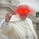2902 뷰
An indicator that multiply the closing price with the current volume . (close X volume )

This will show the relative interest in the underlying asset regardless of the price change over time. For the case of FXCM, when the price fell from \$16 to \$1, its volume spiked 16x at the same time given the fact that 16x more shares can now be purchased with the same amount of dollar.

Enjoy! and remember to give a thumbs up.
오픈 소스 스크립트

진정한 TradingView의 정신에 따라, 이 스크립트의 작성자는 스크립트를 오픈소스로 게시했기에 거래자들이 이해하고 확인할 수 있습니다. 작가님께 건배! 스크립트를 무료로 사용할 수 있지만, 게시물에서 이 코드를 재사용하는 것은 하우스룰을 따릅니다. 당신은 스크립트를 차트에 사용하기 위해 그것을 즐겨찾기 할 수 있습니다.

차트에 이 스크립트를 사용하시겠습니까?
```study("dollar normalized volume", shorttitle="dollar normalized volume")

getVolume(closed, volumed) => closed * volumed

val = getVolume(close, volume)

// draw one that's 3x the price, so we can fake that bars to half itself
//plot(val * 1.5, title='Volume (Invisible)', color=black, transp=100, linewidth=0, style=columns)
plot(val, title='Volume', color=gray, transp=0, linewidth=0, style=columns)
```
may the light of bitcoin be with you

## 코멘트Do uncomment out the following line:

'plot(val * 1.5, title='Volume (Invisible)', color=black, transp=100, linewidth=0, style=columns)'

if you're looknig to display it on the main chart itself:

응답